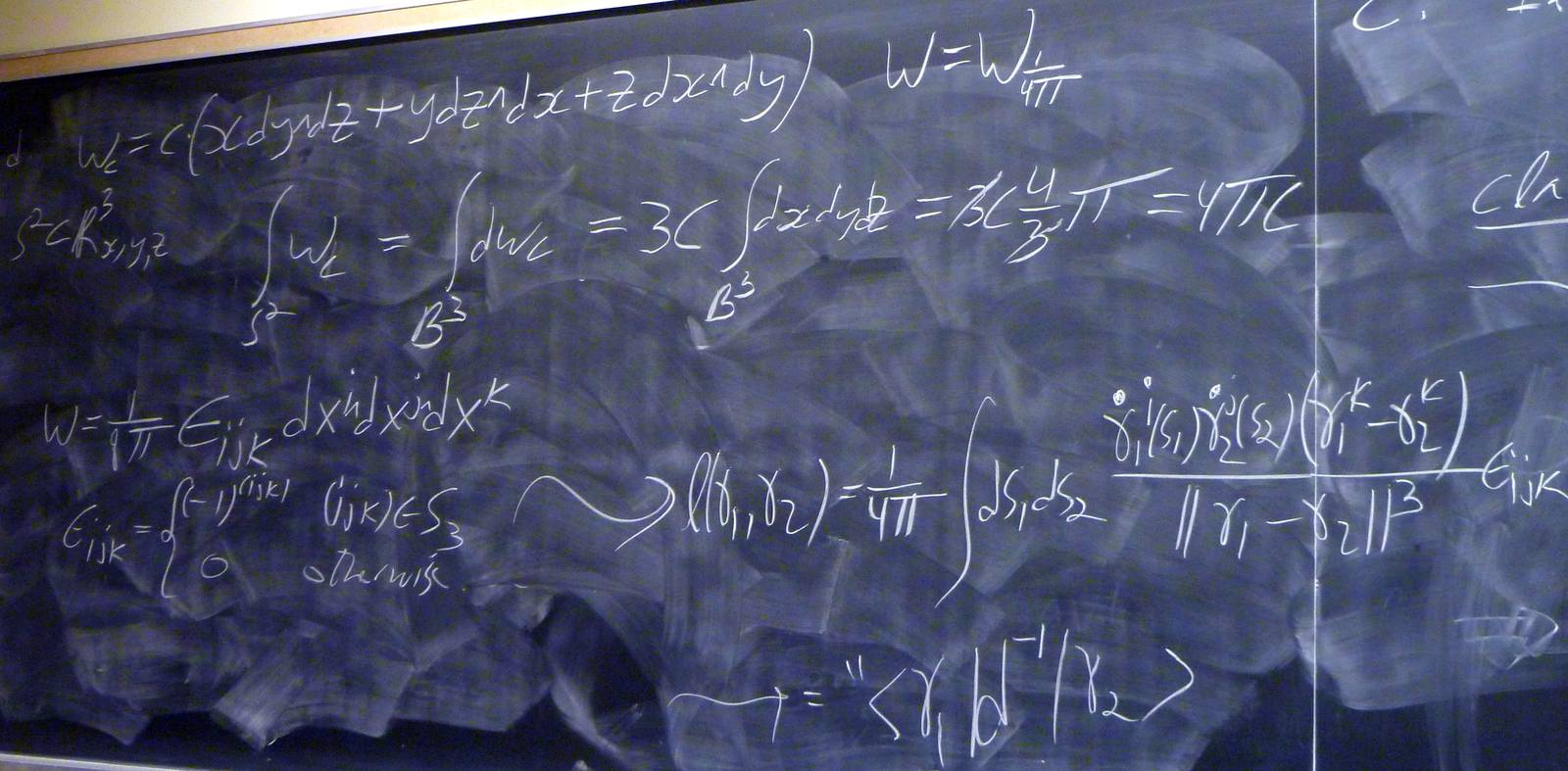© | Dror Bar-Natan: Classes: 2013-14: AKT: < >

# AKT-140108 Video

 width: 400 720 orig/AKT-140108.MOD For now, this video can only be viewed with web browsers that support

Notes on AKT-140108:    [edit, refresh]

The Gauss linking number combinatorially and as an integral.

# Week of... Notes and Links
1 Jan 6 About This Class (PDF).
: Course introduction, knots and Reidemeister moves, knot colourings.
Tricolourability without Diagrams
: The Gauss linking number combinatorially and as an integral.
: The Schroedinger equation and path integrals.
Friday Introduction (the quantum pendulum)
2 Jan 13 Homework Assignment 1.
: The Kauffman bracket and the Jones polynomial.
: Self-linking using swaddling.
: Euler-Lagrange problems, Gaussian integration, volumes of spheres.
3 Jan 20 Homework Assignment 2.
: The definition of finite-type and some examples.
: The self-linking number and framings.
: Integrating a polynomial times a Gaussian.
Class Photo.
4 Jan 27 Homework Assignment 3.
: Chord diagrams and weight systems.
: Swaddling maps and framings, general configuration space integrals.
: Some analysis of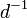$d^{-1}$.
5 Feb 3 Homework Assignment 4.
: 4T, the Fundamental Theorem and universal finite type invariants.
The Fulton-MacPherson Compactification (PDF).
: The Fulton-MacPherson Compactification, Part I.
: More on pushforwards,$d^{-1}$, and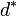$d^\ast$.
6 Feb 10 Homework Assignment 5.
: The bracket-rise theorem and the invariance principle.
: The Fulton-MacPherson Compactification, Part II.
: Gauge fixing, the beginning of Feynman diagrams.
R Feb 17 Reading Week.
7 Feb 24 : A review of Lie algebras.
: Graph cohomology and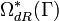$\Omega_{dR}^\ast(\Gamma)$.
: More on Feynman diagrams, beginning of gauge theory.
From Gaussian Integration to Feynman Diagrams (PDF).
8 Mar 3 Homework Assignment 6 (PDF)
: Lie algebraic weight systems.
: Graph cohomology and the construction of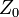$Z_0$.
Graph Cohomology and Configuration Space Integrals (PDF)
: Gauge invariance, Chern-Simons, holonomies.
Mar 9 is the last day to drop this class.
9 Mar 10 Homework Assignment 7 (PDF)
: The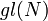$gl(N)$ weight system.
: The universal property, hidden faces.
: Insolubility of the quintic, naive expectations for CS perturbation theory.
10 Mar 17 Homework Assignment 8 (PDF)
: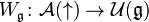$W_{\mathfrak g}\colon{\mathcal A}(\uparrow)\to{\mathcal U}({\mathfrak g})$ and PBW.
: The anomaly.
: Faddeev-Popov, part I.
Gaussian Integration, Determinants, Feynman Diagrams (PDF).
11 Mar 24 Homework Assignment 9 (PDF)
: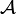${\mathcal A}$ is a bi-algebra.
: Understanding and fixing the anomaly.
Friday: class cancelled.
12 Mar 31 Monday, Wednesday: class cancelled.
: A Monday class: back to expansions.
E Apr 7 : A Friday class on what we mostly didn't have time to do.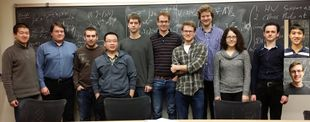Add your name / see who's in!
Dror's Notebookrefresh
panel
Managed by dbnvp: Click the "h:mm:ss" links on the right panel to jump to a specific video time.0:08:12  18S-AKT Question: Why is this sum divisible by 2? Why the $\frac{1}{2}$?

Attempt 1 The factor $\frac{1}{2}$ I think is as a result of the projection of the link unto the plane making each sign appear twice.

Jordan Curve Theorem. If $C$ is a simple closed curve in $\mathbb{R}^2$, then the complement ${\mathbb R}^2\setminus C$ has two components, the interior and the exterior, with $C$ the boundary of each.

Attempt 2The Jordan curve theorem implies that two distinct components in a diagram for a link $L$ intersect an even number of times. Hence we add up an even number of $\pm 1$'s in the computation of $lk(L)$, which yields an even number. It is always an integer. This is why we have a factor of $\frac12$.

Attempt 3 Improved The Jordan curve theorem requires that we have simple closed curves and in general the components of a link are not simple closed curves. However, in the definition of the linking number, only the crossings between the components count. So we can smooth out crossings that are not between the components and this will not affect the linking number; we will then get components that are simple closed curves. From this argument, we can then apply the Jordan curve theorem to get the desired result.

0:10:56 [add] The linking number as a sum over xings.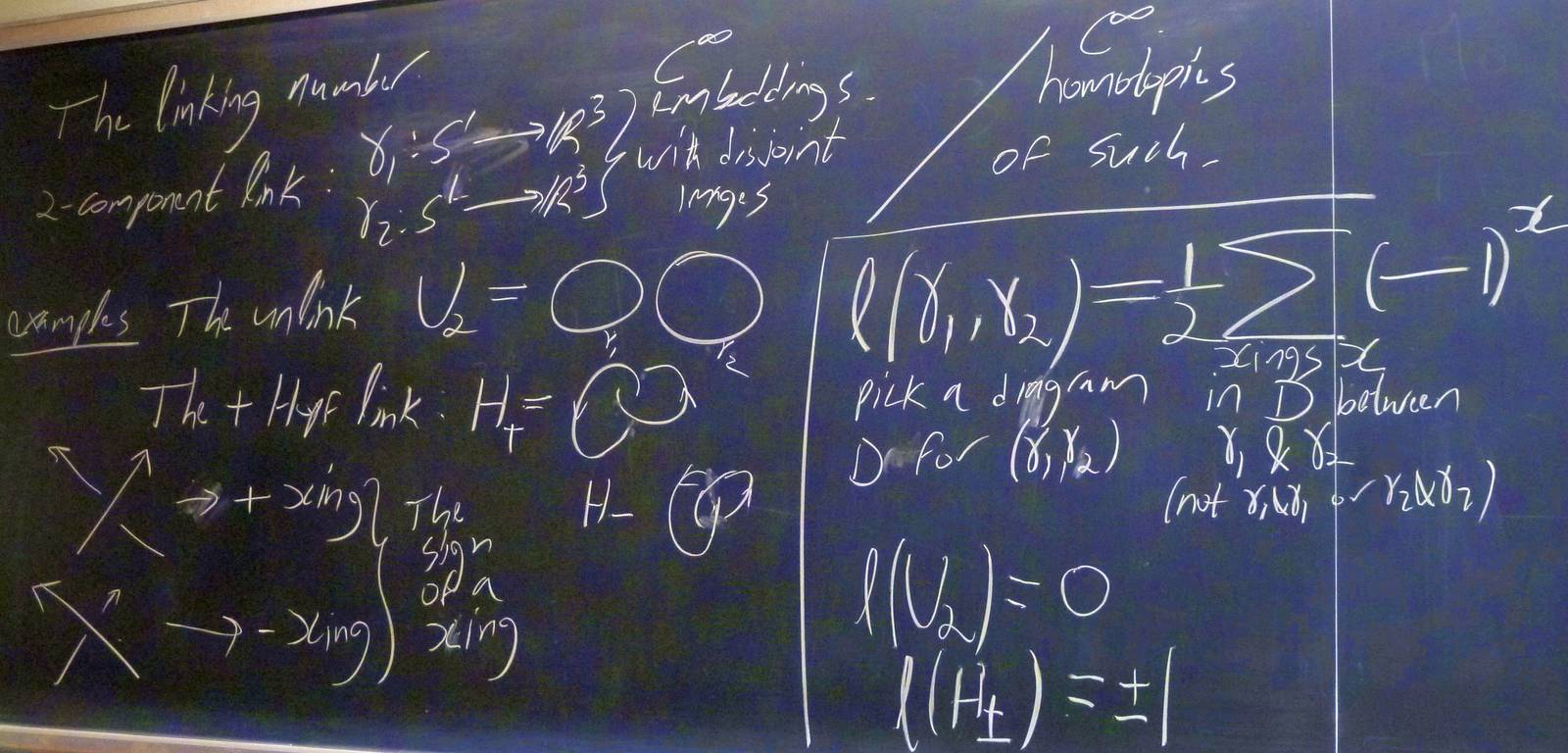0:18:57 [add] Invariance of the linking number sum.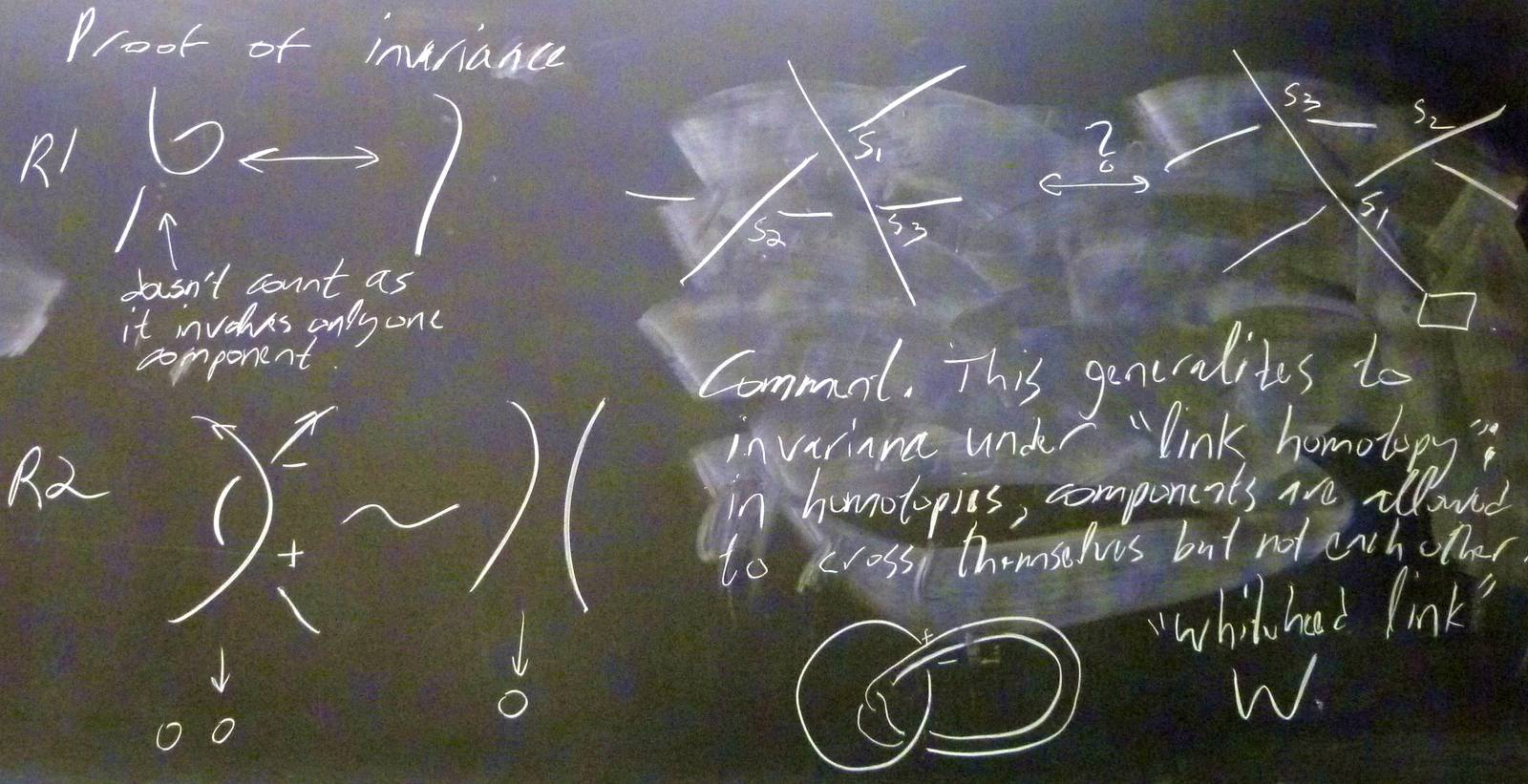0:23:10 [add] The linking number as an integral.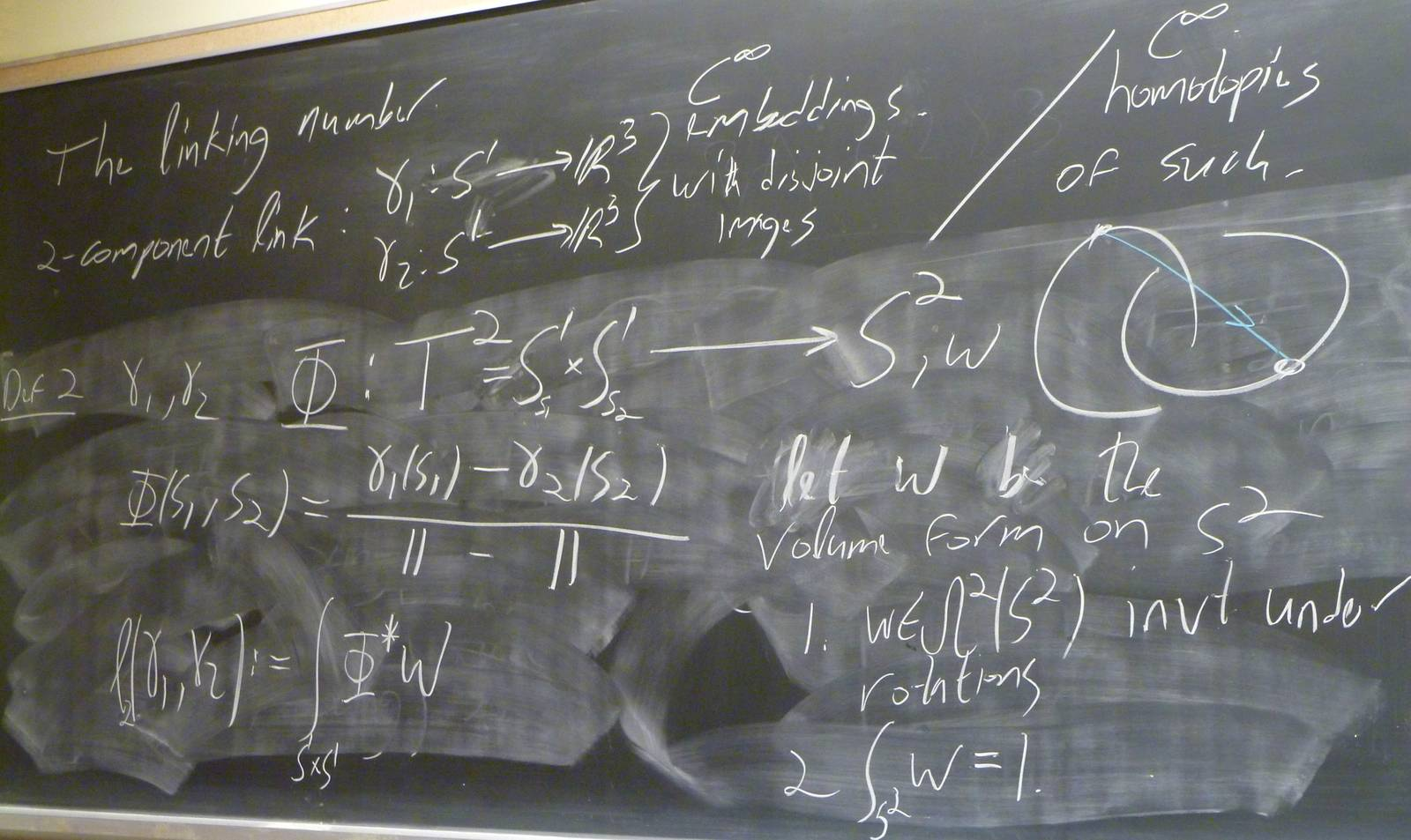0:24:50  I am still not clear on why the image of the unlink is the equator of the sphere. I am wandering why not any other circle above or below the equator. Do we homotop the image to the equator?

Since we have assumed that the embedding is invariant, we can keep the two circles in the same plane. Then, the directional vector would always be inside of the plane. Since we are free to choose which plane we want the unlink circles to be, without loss of generality, the two circles are chosen to be in the xy-plane. Then the directional vector will not have the z-component, hence the image of the map can only be on the equator (if we choose some other planes, the image would be a great circle on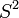$S^2$ by rotating the equator).

0:34:01 [add] Computing the linking number integral.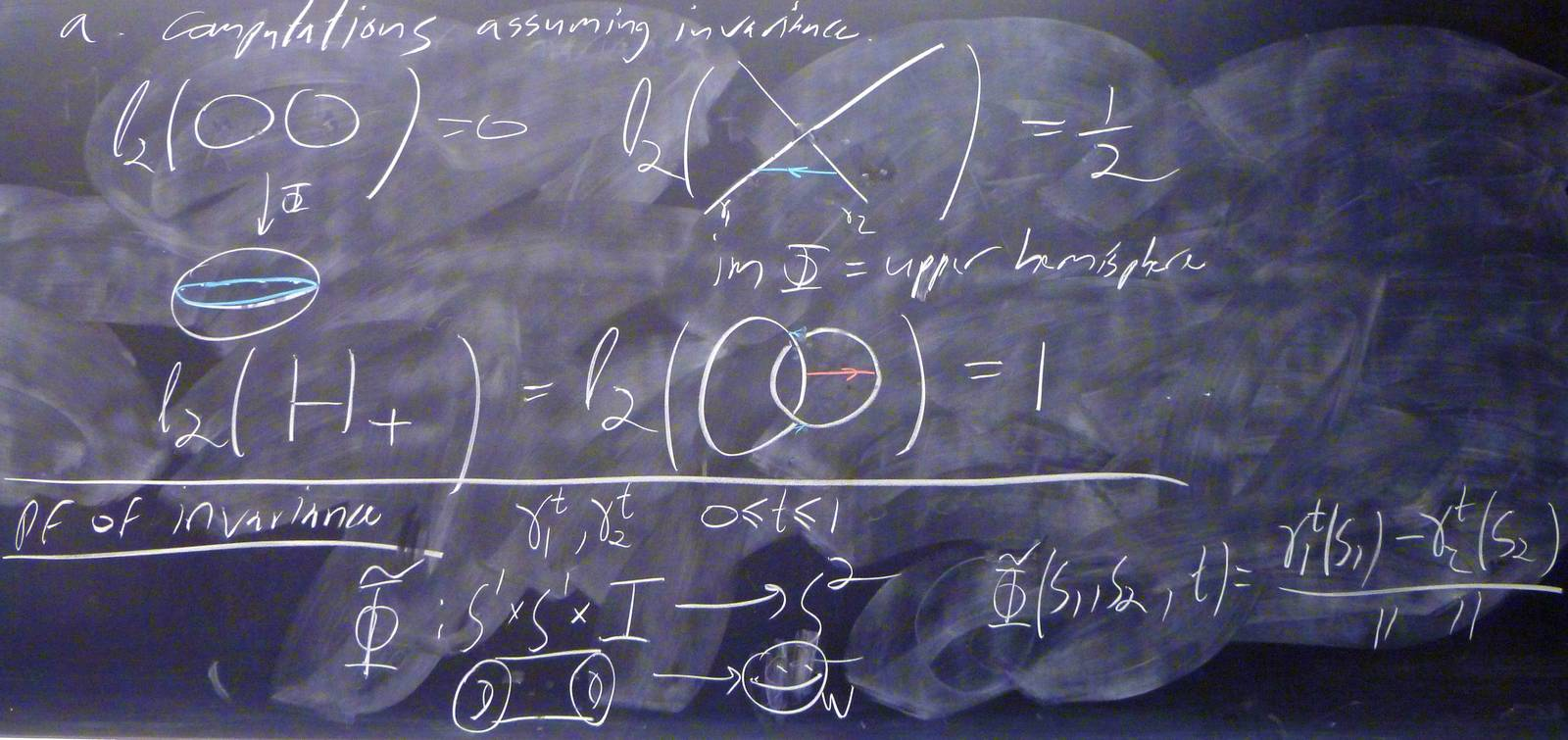0:36:25 [add] Invariance of the linking number integral.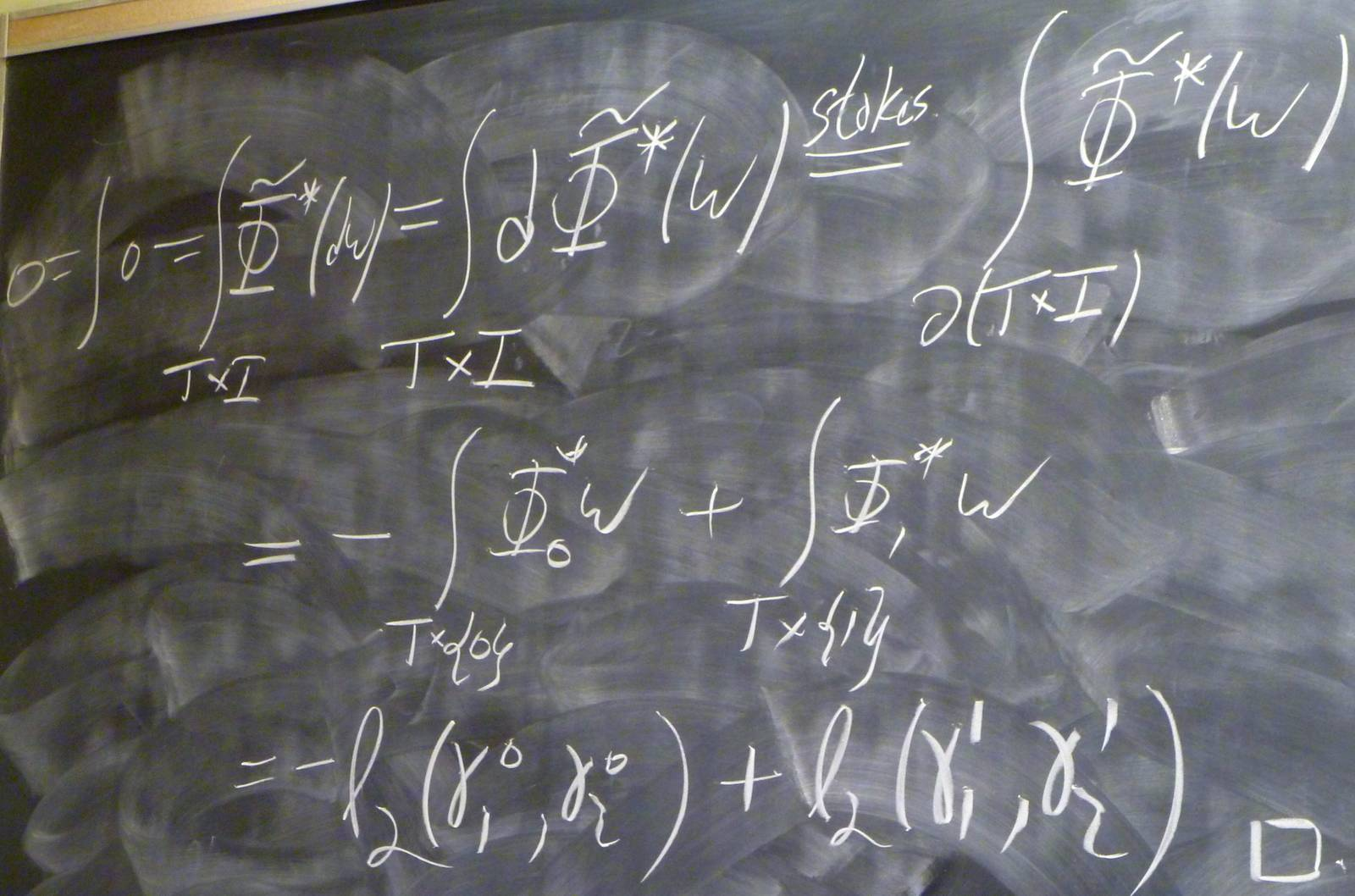0:42:40 [add] Alternative choice of volume forms.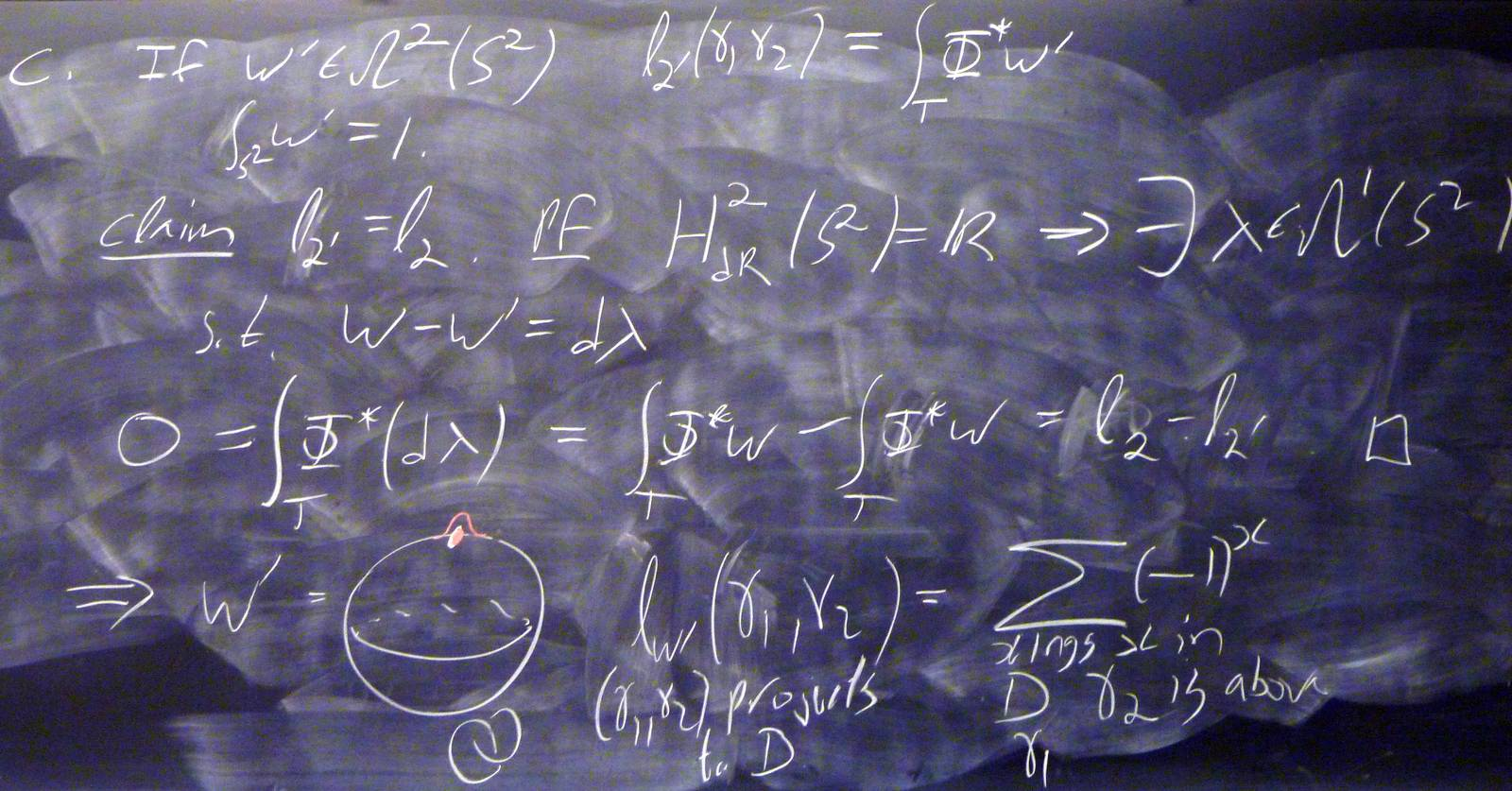0:49:08 [add] An ugly explicit formula for the linking number integral.